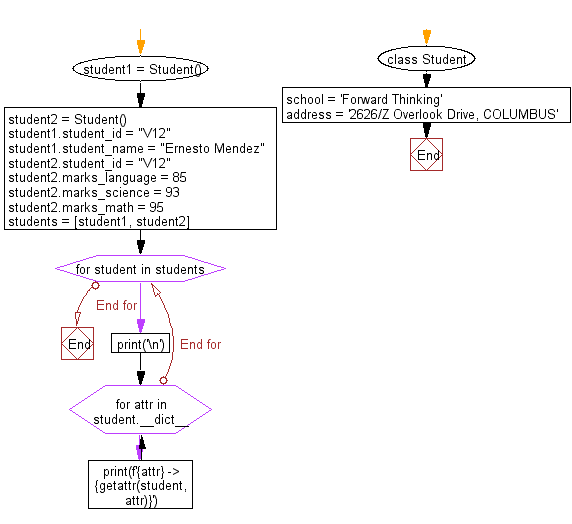﻿ Python: Print all the attributes and their values of instances - w3resource# Python: Print all the attributes and their values of instances

## Python Class ( Basic ): Exercise-12 with Solution

Write a Python class named Student with two instances student1, student2 and assign given values to the said instances attributes. Print all the attributes of student1, student2 instances with their values in the given format.

Input values of the instances:
student_1:
student_id = "V12"
student_name = "Ernesto Mendez"
student_2:
student_id = "V12"
marks_language = 85
marks_science = 93
marks_math = 95
Expected Output:
student_id -> V12
student_name -> Ernesto Mendez
student_id -> V12
marks_language -> 85
marks_science -> 93
marks_math -> 95

Sample Solution:

Python Code:

``````class Student:
school = 'Forward Thinking'
address = '2626/Z Overlook Drive, COLUMBUS'
student1 = Student()
student2 = Student()
student1.student_id = "V12"
student1.student_name = "Ernesto Mendez"
student2.student_id = "V12"
student2.marks_language = 85
student2.marks_science = 93
student2.marks_math = 95
students = [student1, student2]
for student in students:
print('\n')
for attr in student.__dict__:
print(f'{attr} -> {getattr(student, attr)}')
```
```

Sample Output:

```student_id -> V12
student_name -> Ernesto Mendez

student_id -> V12
marks_language -> 85
marks_science -> 93
marks_math -> 95
```

Flowchart:## Visualize Python code execution:

The following tool visualize what the computer is doing step-by-step as it executes the said program:

Python Code Editor:

What is the difficulty level of this exercise?

Test your Programming skills with w3resource's quiz.

﻿

## Python: Tips of the Day

The Zip() Function:

```>>> students = ('John', 'Mary', 'Mike')
>>> ages = (15, 17, 16)
>>> scores = (90, 88, 82, 17, 14)
>>> for student, age, score in zip(students, ages, scores):
...     print(f'{student}, age: {age}, score: {score}')
...
John, age: 15, score: 90
Mary, age: 17, score: 88
Mike, age: 16, score: 82
>>> zipped = zip(students, ages, scores)
>>> a, b, c = zip(*zipped)
>>> print(b)
(15, 17, 16)
```